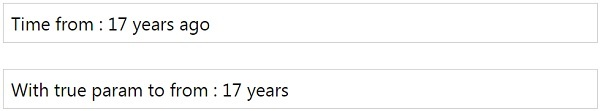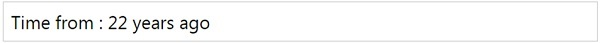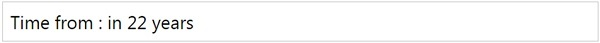# MomentJS - Time from X

This method will tell the length of time from another moment.

## Syntax

```moment().from(Moment|String|Number|Date|Array);
moment().from(Moment|String|Number|Date|Array, Boolean);
```

## Example

```var a = moment([1998, 10, 01]);
var b = moment([2015, 10, 01]);
var c = a.from(b);
var d = a.from(b, true);
```

OR

## Example

```var a = moment([1998, 10, 01]).from([2015, 10, 01]);
var b = moment([1998, 10, 01]).from([2015, 10, 01], true);
```

OR

## Example

```var a = moment([1998, 10, 01]);
var b = a.from([2015, 10, 01], true);
```

## OutputYou can also use JavaScript date as shown in the example given below −

## Example

```var a = moment([1995, 10, 01]).from(new Date());
```

## OutputIf the date given to from is less than the checked date, it gives the output with in instead of ago. Look at the following example for a better understanding −

## Example

```var a = moment(new Date()).from([1995, 10, 01]);
```

## Outputmomentjs_formatting_date_and_time.htm